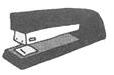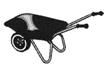Courses

# Test: Work, Force, Energy And Simple Machines - 1

## 10 Questions MCQ Test Science Class 5 | Test: Work, Force, Energy And Simple Machines - 1

Description
This mock test of Test: Work, Force, Energy And Simple Machines - 1 for Class 5 helps you for every Class 5 entrance exam. This contains 10 Multiple Choice Questions for Class 5 Test: Work, Force, Energy And Simple Machines - 1 (mcq) to study with solutions a complete question bank. The solved questions answers in this Test: Work, Force, Energy And Simple Machines - 1 quiz give you a good mix of easy questions and tough questions. Class 5 students definitely take this Test: Work, Force, Energy And Simple Machines - 1 exercise for a better result in the exam. You can find other Test: Work, Force, Energy And Simple Machines - 1 extra questions, long questions & short questions for Class 5 on EduRev as well by searching above.
QUESTION: 1

Solution:
QUESTION: 2

Solution:
QUESTION: 3

### A simple machine

Solution:
QUESTION: 4

Movement of water in ocean due to heating can be shown by the model of

Solution:
QUESTION: 5

Stapler belongs to which class of leverSolution:

Typical staplers are a third-class lever.

QUESTION: 6

A ramp is a good example of

Solution:
QUESTION: 7

A dry comb after combing the hairs is brought near the tiny pieces of paper, what happens?

Solution:
QUESTION: 8

Which of the following form of energy is required to boil the water?

Solution:

QUESTION: 9

(i) Scissors (ii) bottle opener (iii) nail cutter, (iv) forceps. Which of the followings are example of levers.

Solution:
QUESTION: 10

In wheel barrow, which two simple machine mechanism is utilized to make the work easier.Solution: